Author: Oscar Cronquist Article last updated on January 10, 2023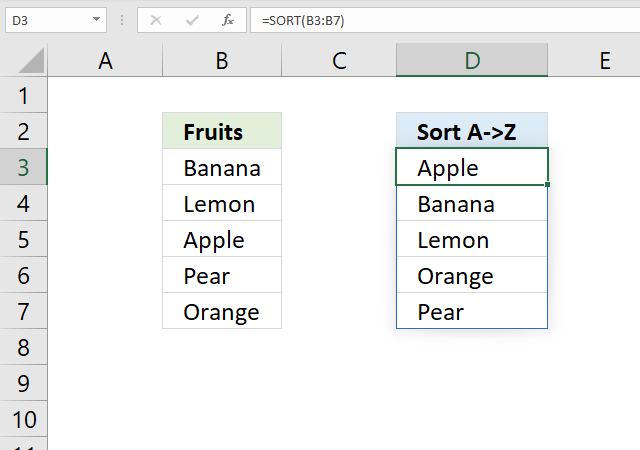The SORT function lets you sort values from a cell range or array. It returns an array with a size that matches the number of values in the array argument.

The SORT function is in the Lookup and reference category and is only available to Excel 365 subscribers.

## 1. SORT Function Syntax

SORT(array, [sort_index], [sort_order], [by_col])

## 2. SORT Function Arguments

 Argument Text array Required. Cell range or array. [sort_index] Optional. A number representing the row / column to sort by. [sort_order] Optional. 1 - Ascending order (A to Z or small to large) -1 - descending order (Z to A or large to small). 1 is the default value if the argument is not specified. [by_col] Optional. False - Sort horizontally (by row), True - Sort vertically (by column). False is the default value if the argument is not specified.

## 3. SORT Function exampleFormula in cell D3:

=SORT(B3:B7)

The formula above sorts the data in cell range C3:C7 and returns the sorted array in cell D3.

## 4. What is a spilled array formula?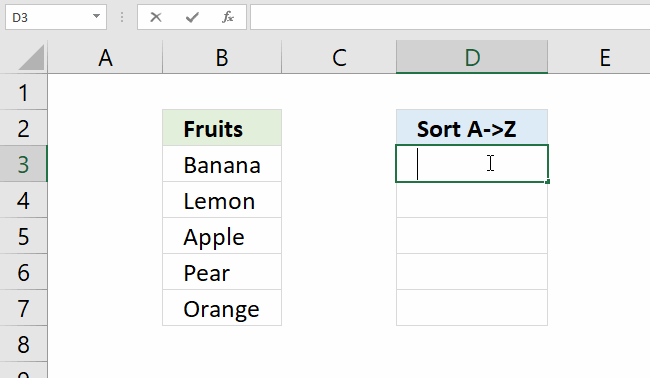A spilled array formula is a formula that returns multiple values. It returns automatically all values to cells below or to the right, this is a new feature for Excel 365 subscribers.

There is no need to enter the formula as an array formula as before, simply press Enter like a regular formula.

The animated image above shows the SORT function being entered in cell D3, as soon as I press Enter the formula returns an array of values to cells below as far as needed.

## 5. Why does the SORT function return a #SPILL! error?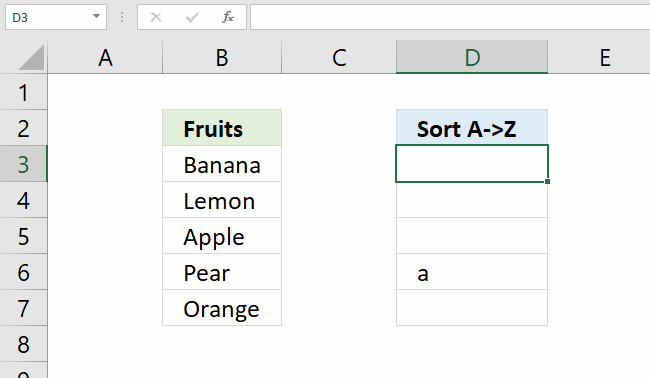A #SPILL! error is returned if one or more cells below are populated. The animated image above shows text value "a" in cell D6.

The SORT function in cell D3 needs cells below to show all values. Cell D6 makes this impossible and a #SPILL! error is returned in cell D3.

You have two options, delete the value in cell D6 or enter the SORT function in another cell that has empty cells below.

## 6. How to sort a specific column in a data set?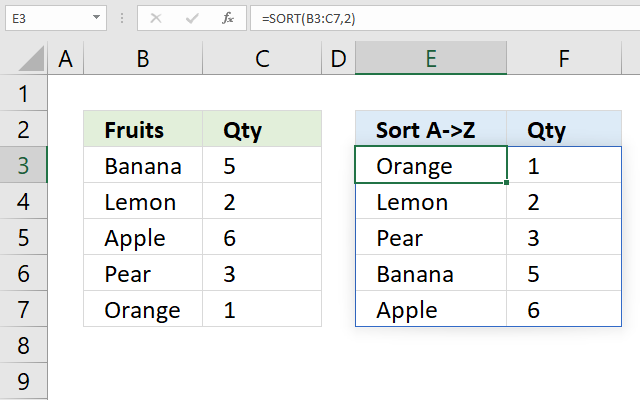The SORT function can sort a multi-column cell range, however, you can only choose one column to sort by. Use the SORTBY function if you need to sort by two or more columns.

Formula in cell E3:

=SORT(B3:C7, 2)

SORT(array, [sort_index], [sort_order], [by_col])

array - B3:C7

[sort_index] - 2

The formula in cell E3 sorts values in cell range B3:C7 based on the second column (column C) from small to large.

## 7. Is it possible to sort letters and numbers?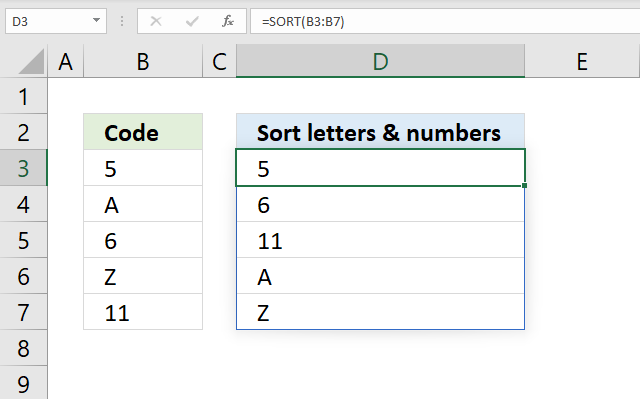Yes, the SORT function sorts the numbers first and then letters if you sort from A to Z.

Formula in cell D3:

=SORT(B3:B7)

## 8. Sort based on an adjacent column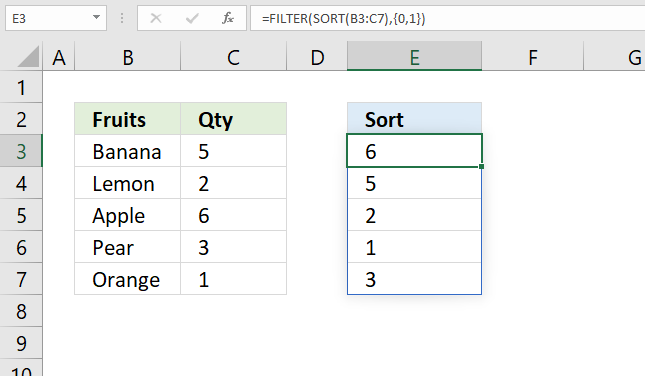The image above demonstrates a formula that sorts values based on cell range B3:C7 by column 1 (B3:B7), however, it returns only column 2 (C3:C7).

Formula in cell E3:

=FILTER(SORT(B3:C7), {0,1})

Here is how the formula works.

#### Step 1 - Sort values from A to z

The SORT function sorts a cell range by the first column from A to Z with the default settings.

SORT(B3:C7)

becomes

SORT({"Banana", 5; "Lemon", 2; "Apple", 6; "Pear", 3; "Orange", 1})

and returns

{"Apple", 6; "Banana", 5; "Lemon", 2; "Orange", 1; "Pear", 3}

#### Step 2 - Extract the second column

The FILTER function can extract the second column using an array containing 0 (zero) and 1. The array must be the same size as the tnumber of columns in the cell range.

1 means that the column is extracted and 0 (zero) means that the column is not extracted.

FILTER(SORT(B3:C7), {0,1})

becomes

FILTER({"Apple", 6; "Banana", 5; "Lemon", 2; "Orange", 1; "Pear", 3}, {0,1})

and returns

{6; 5; 2; 1; 3}

## 9. Is the SORT function case sensitive?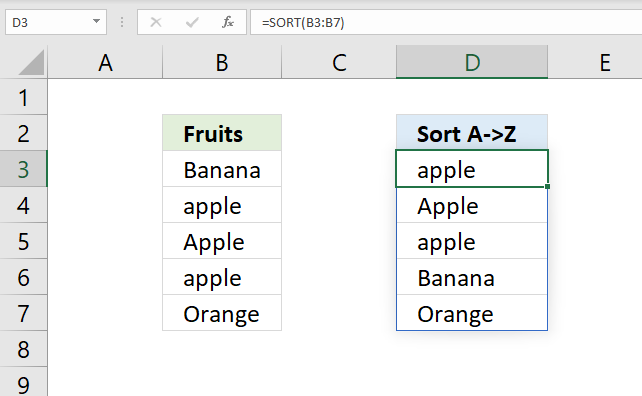No, it is not sorting data based on upper and lower letters. The image above shows that item "apple" and "Apple" with a capital letter is sorted in random order.

## 10. Can you sort data based on an Excel Table?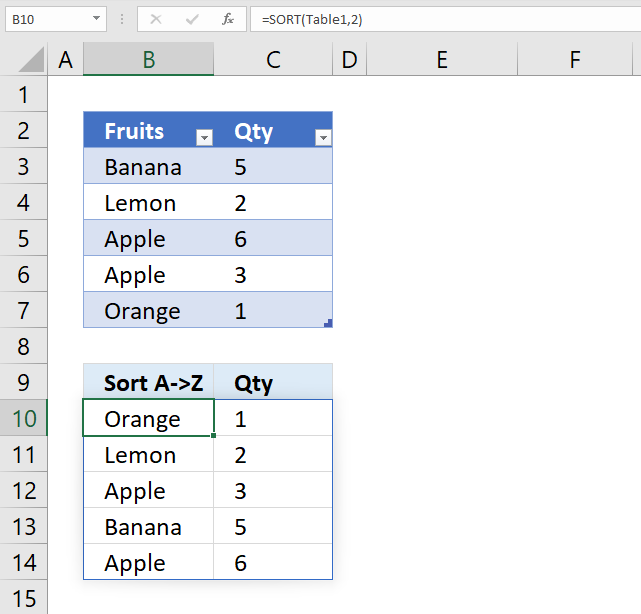Yes, you can. Structured references work fine. Add, delete or edit values in the Excel Table and the SORT function output is instantly changed.

Note, the SORT function returns all values even if the Excel Table is filtered.

## 11. How to sort by column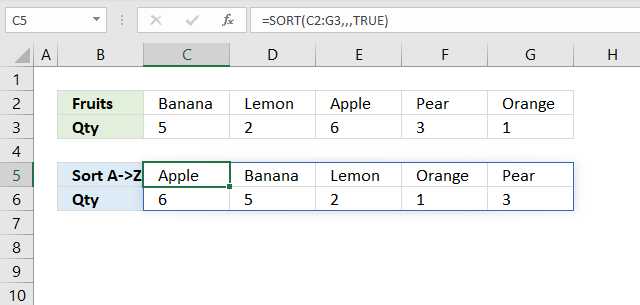The SORT function sorts an array by row if the argument is left out, however, if you use TRUE the SORT function sorts the array by column.

This is demonstrated in the image above.

Formula in cell E3:

=SORT(C2:G3, , , TRUE)

Here is the SORT function syntax:

SORT(array, [sort_index], [sort_order], [by_col])

The last argument [by_col] lets you change the SORT method to by column. TRUE - By column, FALSE or omitted - By row.

## 12. How to sort by column header?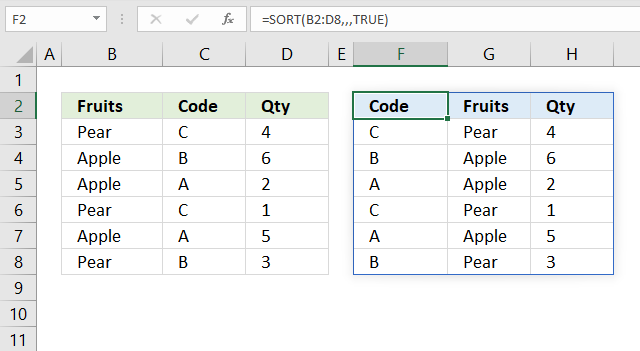You can use the fourth argument [by_col] to sort a cell range by column header. The image above shows the formula in cell F2, the output is sorted based on column header names.

SORT(array, [sort_index], [sort_order], [by_col])

Formula in cell F2:

=SORT(B2:D8,,,TRUE)

You can also sort the column headers from Z to A using this formula:

=SORT(B2:D8,,-1,TRUE)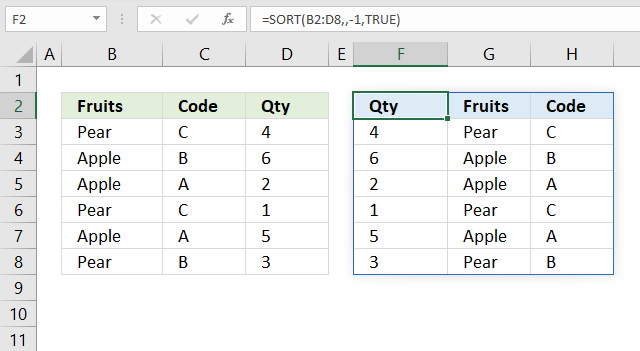## 13. Sum numbers based on items and return totals sorted from large to small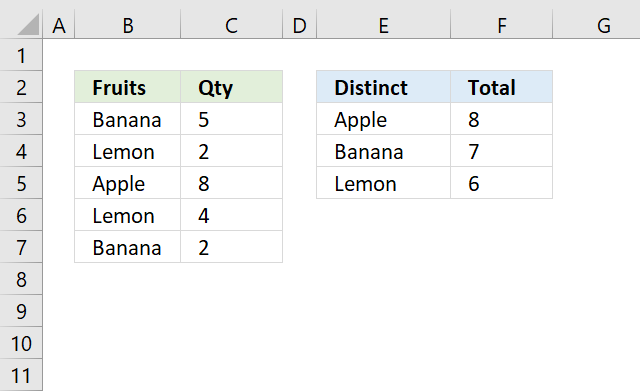The formula in cell F3 adds adjacent numbers based on distinct values and returns totals sorted from large to small. For example, item "Banana" is shown both in cell B3 and B7. The corresponding values are 5 and 2, the total is 7.

This calculation is made for all values in cell range B3:C7, the formula returns the totals for each item sorted from large to small in cell range F3:F5.

Formula in cell F3:

=SORT(SUMIF(B3:B7, UNIQUE(B3:B7), C3:C7), , -1)

Here is how the formula works.

#### Step 1 - Extract unique distinct values from cell range B3:B7

The UNIQUE function returns all distinct values meaning all duplicates are merged into one distinct value.

UNIQUE(B3:B7)

becomes

UNIQUE({"Banana"; "Lemon"; "Apple"; "Lemon"; "Banana"})

and returns

{"Banana"; "Lemon"; "Apple"}

The SUMIF function sums values based on a condition. In this example all distinct values from cell range B3:B7 will be used as criteria.

SUMIF(rangecriteria, [sum_range])

SUMIF(B3:B7, UNIQUE(B3:B7), C3:C7)

becomes

SUMIF(B3:B7, {"Banana"; "Lemon"; "Apple"}, C3:C7)

becomes

SUMIF({"Banana"; "Lemon"; "Apple"; "Lemon"; "Banana"}, {"Banana"; "Lemon"; "Apple"}, {5; 2; 8; 4; 2})

and returns

{7; 6; 8}

#### Step 3 - Sort numbers from large to small

SORT(SUMIF(B3:B7, UNIQUE(B3:B7), C3:C7), , -1)

becomes

SORT({7; 6; 8}, , -1)

and returns

{8; 7; 6}

### Formula in cell E3The formula in cell E3 returns the distinct values sorted based on their total shown in column F.

=SORTBY(UNIQUE(B3:B7),SUMIF(B3:B7,UNIQUE(B3:B7),C3:C7),-1)

#### Step 1 - Extract distinct values

The UNIQUE function returns all distinct values meaning all duplicates are merged into one distinct value.

UNIQUE(B3:B7)

becomes

UNIQUE({"Banana"; "Lemon"; "Apple"; "Lemon"; "Banana"})

and returns

{"Banana"; "Lemon"; "Apple"}.

#### Step 2 - Calculate totals based on distinct values

The SUMIF function sums values based on a condition. In this example, all distinct values from cell range B3:B7 will be used as criteria.

SUMIF(rangecriteria, [sum_range])

SUMIF(B3:B7, UNIQUE(B3:B7), C3:C7)

becomes

SUMIF(B3:B7, {"Banana"; "Lemon"; "Apple"}, C3:C7)

becomes

SUMIF({"Banana"; "Lemon"; "Apple"; "Lemon"; "Banana"}, {"Banana"; "Lemon"; "Apple"}, {5; 2; 8; 4; 2})

and returns

{7; 6; 8}.

#### Step 3 - Sort distinct values based on totals

The SORTBY function sorts an array or cell range, it has the following syntax:

SORTBY(array, by_array1, [sort_order1], [by_array2, sort_order2],…)

SORTBY(UNIQUE(B3:B7), SUMIF(B3:B7, UNIQUE(B3:B7), C3:C7), -1)

becomes

SORTBY(UNIQUE(B3:B7), {7; 6; 8}, -1)

becomes

SORTBY({"Banana"; "Lemon"; "Apple"}, {7; 6; 8},-1)

and returns

{"Apple"; "Banana"; "Lemon"}.

This article demonstrates a formula for earlier Excel versions: Extract unique distinct values sorted based on sum of adjacent values

I recommend a pivot table if you are working with lots of data: Discover Pivot Tables – Excels most powerful feature and also least known

## 14. Sort based on item count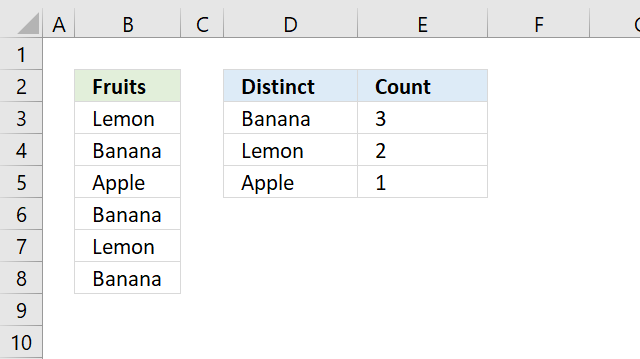The formula in cell E3 calculates the count for each distinct value and sorts the result from large to small. Item "Banan" is shown in cell B4, B6, and B8, the total count is 3 in cell range B3:B8.

The formula in cell D3 returns each distinct value from cell range B3:B8 based on their count from large to small.

Formula in cell E3:

=SORT(COUNTIF(B3:B8, UNIQUE(B3:B8)), , -1)

Here is how the formula works.

#### Step 1 - Extract distinct values

The UNIQUE function returns all distinct values meaning all duplicates are ignored, only one instance of each value is extracted.

UNIQUE(B3:B8)

becomes

UNIQUE({"Lemon"; "Banana"; "Apple"; "Banana"; "Lemon"; "Banana"})

and returns

{"Lemon"; "Banana"; "Apple"}.

#### Step 2 - Count distinct values in cell range B3:B8

The COUNTIF function counts the number of cells that is equal to a condition or criteria.

COUNTIF(range, criteria)

COUNTIF(B3:B8, UNIQUE(B3:B8))

becomes

COUNTIF({"Lemon"; "Banana"; "Apple"; "Banana"; "Lemon"; "Banana"}, {"Lemon"; "Banana"; "Apple"})

and returns

{2; 3; 1}.

#### Step 3 - Sort the result from large to small

The SORT function sorts the array of numbers from large to small.

SORT(array, [sort_index], [sort_order], [by_col])

The second argument [sort_index] allows you to sort the array from large to small if you set it to -1.

SORT(COUNTIF(B3:B8, UNIQUE(B3:B8)), , -1)

becomes

SORT({2; 3; 1}, , -1)

and returns

{3; 2; 1}.

### Formula in cell D3

=SORTBY(UNIQUE(B3:B7), COUNTIF(B3:B8, UNIQUE(B3:B8)), -1)

#### Step 1 - Extract distinct values

The UNIQUE function returns all distinct values meaning all duplicates are ignored.

UNIQUE(B3:B8)

becomes

UNIQUE({"Lemon"; "Banana"; "Apple"; "Banana"; "Lemon"; "Banana"})

and returns

{"Lemon"; "Banana"; "Apple"}.

#### Step 2 - Count distinct values in cell range B3:B8

The COUNTIF function counts the number of cells that is equal to a condition or criteria.

COUNTIF(range, criteria)

COUNTIF(B3:B8, UNIQUE(B3:B8))

becomes

COUNTIF({"Lemon"; "Banana"; "Apple"; "Banana"; "Lemon"; "Banana"}, {"Lemon"; "Banana"; "Apple"})

and returns

{2; 3; 1}.

#### Step 3 - Sort the result from large to small

The SORTBY function sorts an array or cell range, it has the following syntax:

SORTBY(array, by_array1, [sort_order1], [by_array2, sort_order2],…)

SORTBY(UNIQUE(B3:B7),COUNTIF(B3:B8,UNIQUE(B3:B8)),-1)

becomes

SORTBY(UNIQUE(B3:B7),{2; 3; 1},-1)

becomes

SORTBY({"Lemon"; "Banana"; "Apple"},{2; 3; 1},-1)

and returns

{"Banana"; "Lemon"; "Apple"}.

The following article demonstrates a formula for earlier Excel versions: Sort column based on count

## 15. Sort a multicolumn range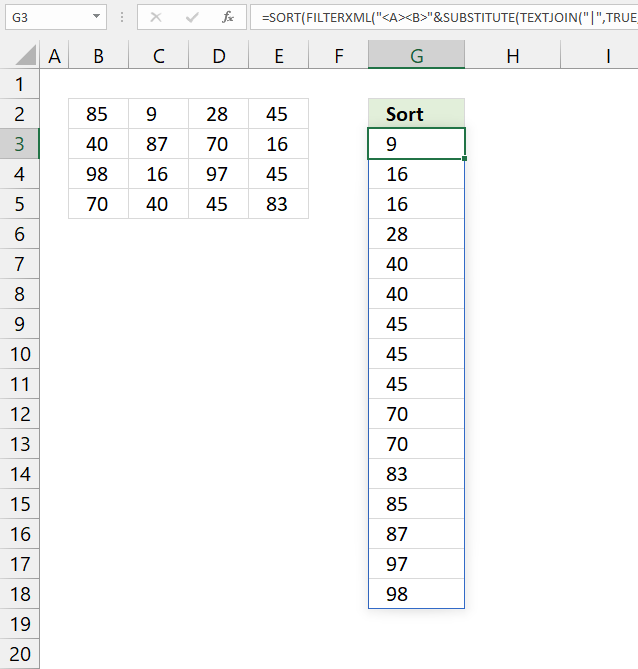This example demonstrates a formula that creates an array of values from a cell range and then sorts the values.

For example, cell range B2:E5 contains numerical values and the SORT function can't sort a multicolumn cell range out of the box.

We need to convert the values to a single column array, to do that we can use the FILTERXML function. The SORT function can now easily sort the values.

Formula in cell G3:

=SORT(FILTERXML("<A><B>"&SUBSTITUTE(TEXTJOIN("|",TRUE,B2:F6),"|","</B><B>")&"</B></A>","//B"))

### Explaining formula in cell G3

#### Step 1 - Join cell values

TEXTJOIN("|",TRUE,B2:F6)

becomes

TEXTJOIN("|", TRUE, {85, 9, 28, 45, 0;40, 87, 70, 16, 0;98, 16, 97, 45, 0;70, 40, 45, 83, 0;0, 0, 0, 0, 0})

and returns

"85|9|28|45|40|87|70|16|98|16|97|45|70|40|45|83"

#### Step 2 - Substitute delimiting character with XML tag

SUBSTITUTE(TEXTJOIN("|",TRUE,B2:F6),"|","</B><B>")

becomes

SUBSTITUTE("85|9|28|45|40|87|70|16|98|16|97|45|70|40|45|83","|","</B><B>")

and returns

"85</B><B>9</B><B>28</B><B>45</B><B>40</B><B>87</B><B>70</B><B>16</B><B>98</B><B>16</B><B>97</B><B>45</B><B>70</B><B>40</B><B>45</B><B>83"

#### Step 3 - Create an array based on XML tags

FILTERXML("<A><B>"&SUBSTITUTE(TEXTJOIN("|",TRUE,B2:F6),"|","</B><B>")&"</B></A>","//B")

becomes

FILTERXML("<A><B>"&"85</B><B>9</B><B>28</B><B>45</B><B>40</B><B>87</B><B>70</B><B>16</B><B>98</B><B>16</B><B>97</B><B>45</B><B>70</B><B>40</B><B>45</B><B>83"&"</B></A>","//B")

becomes

FILTERXML("<A><B>85</B><B>9</B><B>28</B><B>45</B><B>40</B><B>87</B><B>70</B><B>16</B><B>98</B><B>16</B><B>97</B><B>45</B><B>70</B><B>40</B><B>45</B><B>83</B></A>","//B")

and returns {85; 9; 28; 45; 40; 87; 70; 16; 98; 16; 97; 45; 70; 40; 45; 83}.

#### Step 4 - Sort the array

SORT(FILTERXML("<A><B>"&SUBSTITUTE(TEXTJOIN("|",TRUE,B2:F6),"|","</B><B>")&"</B></A>","//B"))

becomes

SORT({85; 9; 28; 45; 40; 87; 70; 16; 98; 16; 97; 45; 70; 40; 45; 83})

and returns {9; 16; 16; 28; 40; 40; 45; 45; 45; 70; 70; 83; 85; 87; 97; 98}.

### Get the Excel fileHow-to-use-the-SORT-function.xlsx